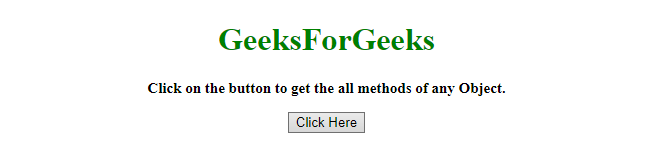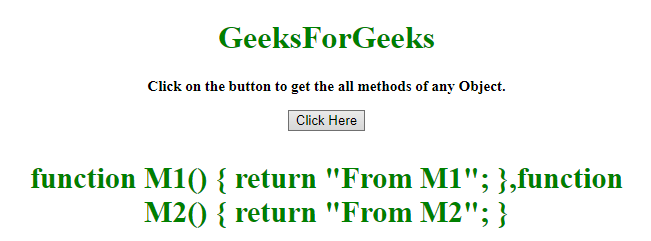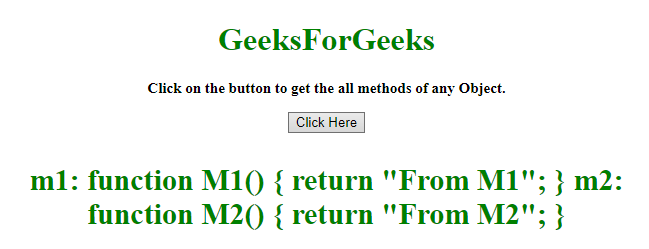Related Articles

# How to get all the methods of an object using JavaScript ?

• Last Updated : 23 Dec, 2019

An HTML document contains some methods and the task is to get all methods of the object. There are two methods to solve this problem which are discussed below:

Approach 1:

• Create a function which takes object as input.
• Use typeof operator, which checks if the type of object is function or not.
• If the type of object is function then it returns the object.

Example: This example implements the above approach.

 ```<``html``>`` ` `<``head``>``    ``<``title``>``        ``Ways to print all methods of an object.``    ```` ` `    ``<``script` `src``=``"https://ajax.googleapis.com/ajax/libs/jquery/3.4.1/jquery.min.js"``>``    `````` ` `<``body` `style``=``"text-align:center;"``>``     ` `    ``<``h1` `style``=``"color: green"``> ``        ``GeeksForGeeks ``    ````     ` `    ``<``p` `id``=``"GFG_UP"` `style``=``        ``"font-size: 15px;font-weight: bold;"``>``    ````     ` `    ``<``button` `onclick``=``"gfg_Run()"``>``        ``Click Here``    ````     ` `    ``<``p` `id``=``"GFG_DOWN"` `style="color:green; ``            ``font-size: 30px; font-weight: bold;">``    ````     ` `    ``<``script``>``        ``var el_up = document.getElementById("GFG_UP");``         ` `        ``var el_down = document.getElementById("GFG_DOWN");``         ` `        ``el_up.innerHTML = "Click on the button to get "``                    ``+ "the all methods of any Object.";`` ` `        ``function Obj() {``            ``this.m1 = function M1() {``                ``return "From M1";``            ``}``            ``this.m2 = function M2() {``                ``return "From M2";``            ``}``        ``}`` ` `        ``function getAllMethods(obj = this) {``            ``return Object.keys(obj)``                ``.filter((key) => typeof obj[key] === 'function')``                ``.map((key) => obj[key]);``        ``}`` ` `        ``function gfg_Run() {``            ``el_down.innerHTML = getAllMethods(new Obj());``        ``}``    `````` ` ``

Output:

• Before clicking on the button:• After clicking on the button:Approach 2:

• Create a function which takes object as input.
• Use typeof operator, which checks if the type of object is function or not. This example also checks if any error occurred or not and if occurred then handle it properly.
• If the typeof Object is function then return it.

Example 2: This example implements the above approach.

 ```<``html``>`` ` `<``head``>``    ``<``title``>``        ``Ways to print all methods of an object.``    ````    ``<``script` `src``=``"https://ajax.googleapis.com/ajax/libs/jquery/3.4.1/jquery.min.js"``>``    `````` ` `<``body` `style``=``"text-align:center;"``      ``id``=``"body"``>``    ``<``h1` `id``=``"h1"``        ``style``=``"color: green"``>  ``            ``GeeksForGeeks  ``        ````    ``<``p` `id``=``"GFG_UP"` `       ``style="font-size: 15px;``              ``font-weight: bold;">``    ````    ``<``button` `onclick``=``"gfg_Run()"``>``        ``Click Here``    ````    ``<``p` `id``=``"GFG_DOWN"` `       ``style="color:green;``              ``font-size: 30px;``              ``font-weight: bold;">``    ````    ``<``script``>``        ``var el_up = ``            ``document.getElementById("GFG_UP");``        ``var el_down = ``            ``document.getElementById("GFG_DOWN");``        ``el_up.innerHTML = ``"Click on the button to get the all methods of any Object.";`` ` `        ``function Obj() {``            ``this.m1 = function M1() {``                ``return "From M1";``            ``}``            ``this.m2 = function M2() {``                ``return "From M2";``            ``}``        ``}`` ` `        ``function getAllMethods(obj) {``            ``var result = [];``            ``for (var id in obj) {``                ``try {``                    ``if (typeof(obj[id]) == "function") {``                        ``result.push(id + ": " + obj[id].toString());``                    ``}``                ``} catch (err) {``                    ``result.push(id + ": Not accessible");``                ``}``            ``}``            ``return result;``        ``}`` ` `        ``function gfg_Run() {``            ``el_down.innerHTML = getAllMethods(new Obj()).join("\n");``        ``}``    `````` ` ``

Output:

• Before clicking on the button:• After clicking on the button:Hey geek! The constant emerging technologies in the world of web development always keeps the excitement for this subject through the roof. But before you tackle the big projects, we suggest you start by learning the basics. Kickstart your web development journey by learning JS concepts with our JavaScript Course. Now at it’s lowest price ever!

My Personal Notes arrow_drop_up